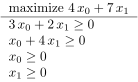# A question of presentation

Here is a code which works as expected

nl=3
nc=2
ring=QQ
A=matrix(ring,nl,nc,[3,2,1,4,1,1])
b=vector(ring,[3,5,6])
c=vector(ring,[4,7])
sign=list([">=",">=","<="])
vsign=list([">=",">="])
show(LatexExpr(r"\boldsymbol{A}="),A)
show(LatexExpr(r"\boldsymbol{b}="),b)
show(LatexExpr(r"\boldsymbol{c}="),c)
show(LatexExpr(r"\boldsymbol{Signs}="),sign)
show(LatexExpr(r"\boldsymbol{Vsigns}="),vsign)

def primal_lp(opt,A,b,c,signAb,signx):
nc=A.ncols()
x = var("x_", n=nc)
X=[xj for xj in x]
Zer1 =[0 for v in x]
Zer2 =[0 for v in range(len(signAb))]
con=A*vector(X)
z=c*vector(X)
from _operator import le, ge, eq
op1=[ge  if v==">=" else (le if v=='<=' else eq)  for v in signx]
tt1=list(map(lambda u,v:u(*v), op1 ,zip(X,Zer1)))
op2=[ge  if v==">=" else (le if v=='<=' else eq)  for v in signAb]
tt2=list(map(lambda u,v:u(*v), op1 ,zip(con,Zer2)))
z1=flatten([opt,z,tt2,tt1])
return show(z1)
primal_lp('max',A,b,c,sign,vsign)


I would like to present the results like

$\max 4 x_0 + 7 x_1$

$3 x_0 + 2 x_1\geq 0$

$x_0+4 x_1 \leq 0$

$x_0+x_1=0$

$x_0≥0$

$x_1≤0$

I have tried to use table but I haven't found any way to obtain the desired representation. Any idea ?

edit retag close merge delete

Sort by » oldest newest most voted

One possible way to do it :

nl=3
nc=2
ring=QQ
A=matrix(ring,nl,nc,[3,2,1,4,1,1])
b=vector(ring,[3,5,6])
c=vector(ring,[4,7])
# Use expressions rather than strings : more useful, less hassle.
# sign=list([">=",">=","<="])
# vsign=list([">=",">="])
from _operator import eq, le, lt, ge, gt
# Don't scratch built-in "sign" function, it may come handy later on
hsign = [ge, ge, le]
vsign = [ge, ge]
# show(LatexExpr(r"\boldsymbol{A}="),A)
# show(LatexExpr(r"\boldsymbol{b}="),b)
# show(LatexExpr(r"\boldsymbol{c}="),c)
# show(LatexExpr(r"\boldsymbol{Signs}="),sign)
# show(LatexExpr(r"\boldsymbol{Vsigns}="),vsign)
# Neither Python's max nor Sage's symbolic_max accept a single argument,
# and neither denote maximization. Let's use an ad hoc symbolic function
maximize = function("maximize",
print_latex_func=lambda self, *args:
r"\text{maximize}\ %s"%latex(args))

def primal_lp(opt,A,b,c,signAb,signx):
nc=A.ncols()
x = var("x_", n=nc)
# X=[xj for xj in x] # This exact copy has no use.
# X replaced by x afterwards...
# Zer1 =[0 for v in x]
Zer1 = *len(x) # faster
# Zer2 =[0 for v in range(len(signAb))]
Zer2 = *len(signAb) # MUCH faster
con=A*vector(x)
# z=c*vector(x) # Used once, put it inline...
# from _operator import le, ge, eq # Done at toplevel
# No neeed since we use expressions
# op1=[ge  if v==">=" else (le if v=='<=' else eq)  for v in signx]
tt1=list(map(lambda u,v:u(*v), signx ,zip(x,Zer1)))
# Ditto
# op2=[ge  if v==">=" else (le if v=='<=' else eq)  for v in signAb]
tt2=list(map(lambda u,v:u(*v), signx ,zip(con,Zer2)))
# z1=flatten([opt,z,tt2,tt1])
# return show(z1)
z1 = [opt(c*vector(x))]+tt2+tt1
# If we want to return a table
# z1=table([[u] for u in tt2+tt1],
return z1


This returns a list of expressions :

sage: primal_lp(maximize,A,b,c,hsign,vsign)
[maximize(4*x_0 + 7*x_1),
3*x_0 + 2*x_1 >= 0,
x_0 + 4*x_1 >= 0,
x_0 >= 0,
x_1 >= 0]


whose tabulation is easy : view(table([[u] for u in primal_lp(maximize,A,b,c,hsign,vsign)])) will give you something like :[ I had to recourse to image conversion, since ask.sagemath.org's damn Mathjax won't accept tabular expressions :

sage: print(latex(table([[u] for u in primal_lp(maximize,A,b,c,hsign,vsign)])))
\begin{tabular}{l}
$\text{maximize}\ 4 \, x_{0} + 7 \, x_{1}$ \\
$3 \, x_{0} + 2 \, x_{1} \geq 0$ \\
$x_{0} + 4 \, x_{1} \geq 0$ \\
$x_{0} \geq 0$ \\
$x_{1} \geq 0$ \\
\end{tabular}


]

HTH,

EDIT : slelievre's suggestion worked (on Sagecell), and gave the following result :

 $\text{maximize}\ 4 \, x_{0} + 7 \, x_{1}$ $3 \, x_{0} + 2 \, x_{1} \geq 0$ $x_{0} + 4 \, x_{1} \geq 0$ $x_{0} \geq 0$ $x_{1} \geq 0$

more

I had to [resort] to image conversion [...]

sage: p = primal_lp(maximize, A, b, c, hsign, vsign)
sage: t = table([[u] for u in p])
sage: print(html(t))
<div class="notruncate">
<table  class="table_form">
<tbody>
<tr class ="row-a">
<td style="text-align:left">$\text{maximize}\ 4 \, x_{0} + 7 \, x_{1}$</td>
</tr>
<tr class ="row-b">
<td style="text-align:left">$3 \, x_{0} + 2 \, x_{1} \geq 0$</td>
</tr>
<tr class ="row-a">
<td style="text-align:left">$x_{0} + 4 \, x_{1} \geq 0$</td>
</tr>
<tr class ="row-b">
<td style="text-align:left">$x_{0} \geq 0$</td>
</tr>
<tr class ="row-a">
<td style="text-align:left">$x_{1} \geq 0$</td>
</tr>
</tbody>
</table>
</div>


Which can be pasted into Ask Sage, and gets rendered as:

 $\text{maximize}\ 4 \, x_{0} + 7 \, x_{1}$ $3 \, x_{0} + 2 \, x_{1} \geq 0$ $x_{0} + 4 \, x_{1} \geq 0$ $x_{0} \geq 0$ $x_{1} \geq 0$

Dear Samuel, your solution is clever for somebody who use a standard Latex distribution including Pdflatex. But I am working exclusively with Sagecell. I do not know if it is possible to call non pre installed packages of Sagemath. Nevertheless, I finally used some of your advices.

On websites rendering Markdown, MathJax is launched on expressions between dollar signs, so it is automatically in math mode. Not sure how to deal with a tabular then, especially a tabular with math expressions inside. But this is maybe getting off-topic here.